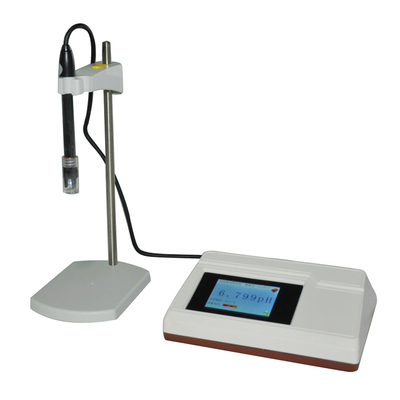客服热线：010-80764046

# 电导率仪测量原理及单位换算解析

1= 102cm(厘)=103m(毫)=106μ(微) 如上面的Ω-1 cm-1=S cm-1=106μS/cm

0.5mS/m=0.5×103μS/(1×102)cm=5μS/cm 二级水≤0.1mS/m=0.1×103μS/(1×102)cm=1μS/cm 即：1 mS/m =10μS/cm 同理：1S/m=103 mS/m =104μS/cm